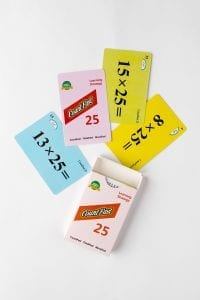# CountFast 25 Lesson Plan

####OVERVIEW & PURPOSE

Our final week of the 3rd Grade CountFast program focuses on specific mental strategies for multiplying with the numbers 25 and 75. Spend 15 minutes each day on one of the activities listed in this module.  Card decks should go home with students each day for additional practice with a parent at home. Each week, a new deck is introduced, and the previous deck is for the student to keep at home for continued practice.

#### EDUCATION STANDARDS

1.    CCSS.Math.Content.3.OA.B.5
Apply properties of operations as strategies to multiply and divide.
2.  NCTM Standard: develop a sense of whole numbers and represent and use them in flexible ways, including relating, composing, and decomposing numbers

#### OBJECTIVES

1. Quickly, mentally multiply by 25 and 75.
2. Develop fluency with multiplication calculations.

#### MATERIALS NEEDED

1. One CountFast 25, 75 card deck for each student.  This deck is for school and home use.  Discuss routine and expectations for taking home the deck and returning it to school each day.
2. One writing utensil per student, optional per teacher plans.

#### CountFast 25, 75 Pack – Day 1 and 2

Teacher Model/Direct:  Use the yellow cards from the deck and teach students the fast way to multiply any number by 25.  Since 25 is one-fourth of 100, we will first multiply the number by 100 (by simply adding two zeros to the end of the number), and then dividing that answer by 4.  For example:

• 8 x 25 = 800 ÷ 4                                         1) 15 x 25 = 1500 ÷ 4               1) 20 x 25 = 2000 ÷ 4
• 800 ÷ 4= 200                                              2) 1500 ÷ 4 = 375                     2) 2000 ÷ 4 = 500

(same as 8 ÷ 4, add back zeros)

Student Activity:  Give each student a deck and ask them to take out the yellow cards.  Working with a partner, take turns holding up the cards for the partner to multiply, using the method learned in class.

Home Activity: Students will take home the deck and the “CountFast Home Connection” letter.  Students will review the yellow cards with a parent and explaining how to solve the problems quickly using the method learned in class. Parent can record how quickly the child multiplies all the cards correctly.

CountFast 25, 75 Pack – Day 3 and 4

Teacher Model/Direct:  Using the same procedure as Day 1, review the strategy for quickly multiplying any number times 25.  Then use the pink cards to introduce how to expand on that strategy to multiply any number by 75.  Since 75 is the same as 3 X 25, first multiply the number by 3, and then use that product and follow the ‘times 25’ procedure learned on Day 1.

For example: 13 x 75

First, we multiply the number by 3:            13 X 3 = 39

Now, we multiply by 100:          39 X 100 = 3900

The last step is to divide by 4, and in order to do so we just divide twice by 2:

3900 / 2 = 1950                                          1950 / 2 = 975

We end up with, 13 x 75 = 975

Student Activity:  Students will work in partners to practice with the pink cards, using the mental calculation tips for multiplying by 75.

Home Activity: Students will repeat the partner practice at home with a parent.

#### Instructional Video

Stay SharpFree flash card decks to keep kids entertained and sharp!

To help you keep your kids math sharp during this crisis, we're giving you 3 free CountFast flash card decks for just \$6 in shipping.  Just have them take the quick quiz for their grade at the link below and you'll get a discount code to use on any three decks.